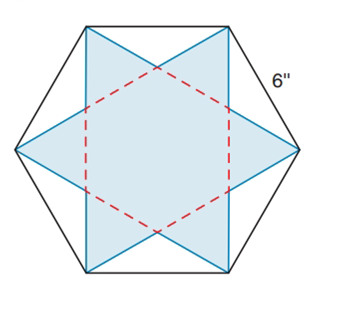Chapter 8.3, Problem 40EElementary Geometry For College St...

7th Edition
Alexander + 2 others
ISBN: 9781337614085

Solutions

Chapter
SectionElementary Geometry For College St...

7th Edition
Alexander + 2 others
ISBN: 9781337614085
Textbook Problem

The length of each side of a regular hexagon measures 6   i n . Find the area of the inscribed regular hexagram shaded in the figure.To determine

To find:

The area of the regular hexagram.

Explanation

Calculation:

Given,

The length of each side of a regular hexagon is 6 in.

Calculation:

Consider the regular hexagon,

Area of the regular hexagon is 332a2, where a is the side length of the hexagon.

given each side of a hexagon is 6. i.e., a=6

Therefore,

AABCDEF=332×62=332×36=33×18AABCDEF=543in2...(1)

Draw a line ON such that ON is perpendicular to ED and N is the midpoint of ED

Since OND is a right angle triangle and the angle of the triangle is 306090.

Therefore by the properties of 306090 triangle the hypotenuse is equal to twice the length of the shorter leg, which is the side across from the 30 angle. The longer leg, which is across from the 60 angle, is equal to multiplying the shorter leg by the 3.

Let ON=x

then OD=2x and ND=3x

given ED=6

which implies ND=62=3.

Substitute ND=3 in ND=3x, we get

3=3x

x=33

x=3

i.e

Still sussing out bartleby?

Check out a sample textbook solution.

See a sample solution

The Solution to Your Study Problems

Bartleby provides explanations to thousands of textbook problems written by our experts, many with advanced degrees!

Get Started

In Exercises 2528. solve the equation by factoring. 26. 6x2 10x + 4 = 0

Applied Calculus for the Managerial, Life, and Social Sciences: A Brief Approach

(a) Prove Theorem 4, part 3. (b) Prove Theorem 4, part 5. Theorem 4

Single Variable Calculus: Early Transcendentals, Volume I

Find the following products. (3+7i)(37i)

Trigonometry (MindTap Course List)

Perform the indicated operation for the following. 5.

Contemporary Mathematics for Business & Consumers